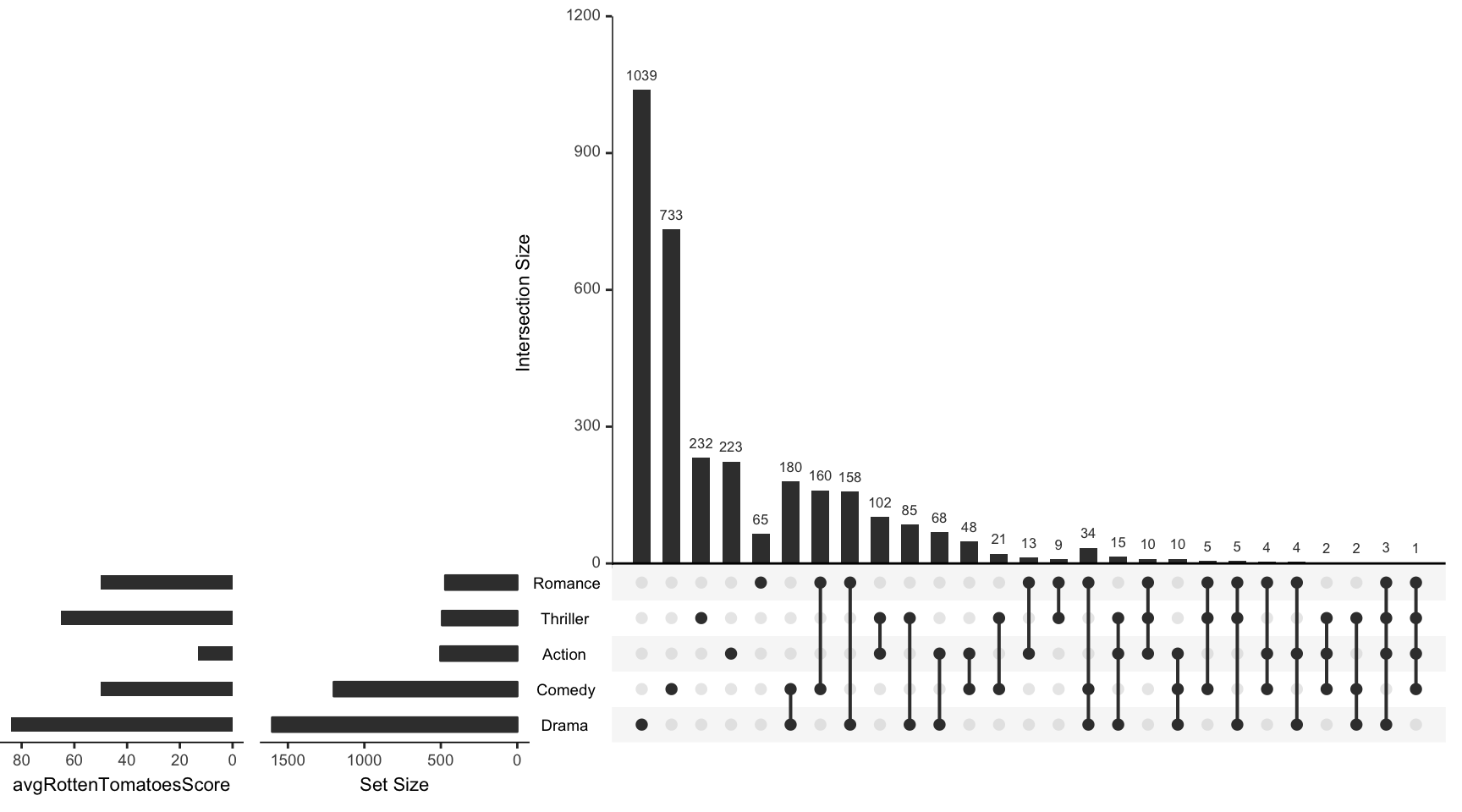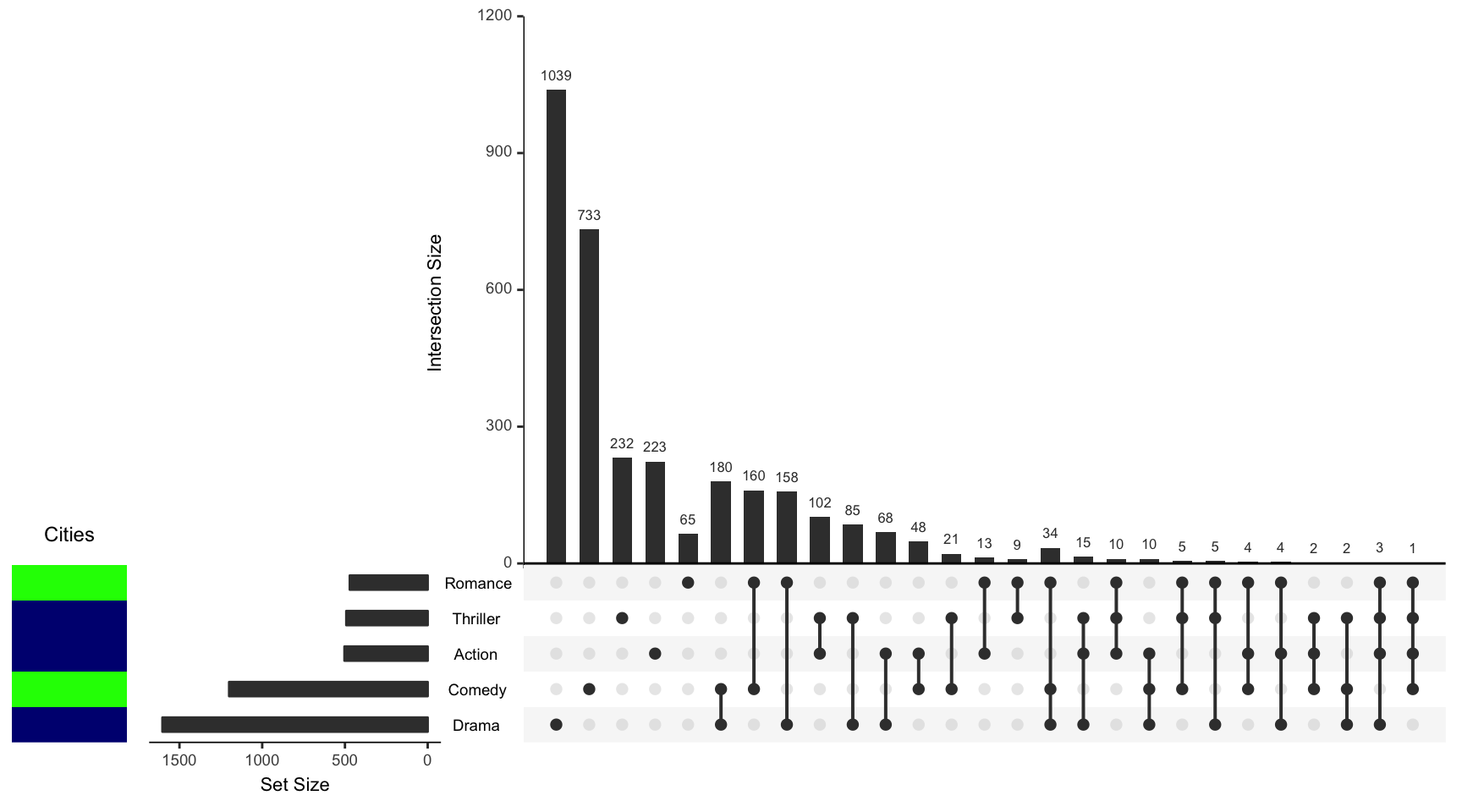For all examples the movies data set contained in the package will be used.

library(UpSetR)
movies <- read.csv(system.file("extdata", "movies.csv", package = "UpSetR"),
header = T, sep = ";")

The set.metadata parameter is broken up into 3 fields: data, ncols, and plots.

• data: takes a data frame where the first column is the set names, and the following columns are attributes of the sets.

• plots: is a list that takes a list of parameters that are used to generate the plots. These parameters include column, type, assign, and colors.

• column: is the column of the dataframe that should be used for the specified plot.

• type: is what type of plot should be used to display the data from the specified column. If the data in the column is numeric, then the plot type can be either a bar plot ("hist"), or heat map ("heat"). If the data in the column is boolean, then the plot type can be a "bool" heat map. If the data in the column is categorical (character), then the plot type can either be a heat map ("heat") or text ("text"). Additionally, if the data in the column is ordinal (factor), then the plot type can be either a heat map or text. There is also a type called "matrix_rows" which allows us to use apply colors to the matrix background using categorical data. This type is useful for identifying characteristics of sets using the matrix.

• assign: is the number of the columns that should be assigned to the specific plot. For instance if you’re plotting 2 set metadata plots then you may choose one plot to take up 20 columns and other plot 10 columns. Since the UpSet plot is typically plotted on a 100 by 100 grid, the grid will now be 100 by 130 where roughly 1/4 ofthe plot is assigned to the metadata plots.

• colors: is used to specify the colors used in the metadata plots. If the plot type is a bar plot then the parameter only takes one color for the whole plot. If the plot type is "heat" or "bool", then a vector of colors can be provided where there is one color for each unique category (character). However, if the data type is ordinal (factor) there is no colors input and the heat map works on a color gradient rather than applying different colors to each level. Lastly, if the plot type is “text" then a vector of colors can be provided where there is one color for each unique string. If not colors are provided, a color palette will be provided for you.

## Example 1: Set Metadata Bar Plot

In this example, the average Rotten Tomatoes movie ratings for each set will be used as the set metadata. This may help us draw more conclusions from the visualization by knowing how professional movie reviewers typically rate movies in these categories.

sets <- names(movies[3:19])
avgRottenTomatoesScore <- round(runif(17, min = 0, max = 90))
names(metadata) <- c("sets", "avgRottenTomatoesScore")

When generating a bar plot using set metadata information it is important to make sure the specified column is numeric.

is.numeric(metadata$avgRottenTomatoesScore) ##  FALSE The column is not numeric! In fact it is a factor, so we must coerce it to characters and then to integers. metadata$avgRottenTomatoesScore <- as.numeric(as.character(metadata$avgRottenTomatoesScore)) upset(movies, set.metadata = list(data = metadata, plots = list(list(type = "hist", column = "avgRottenTomatoesScore", assign = 20))))## Example 2: Set Metadata Heat Map In this example we will make our own data on what major cities these genres were most popular in. Since this is categorical and not ordinal we must remember to change the column to characters (it is a factor again). To make sure we assign specific colors to each category you can specify the name of each category in the color vector, as shown below. If you don’t care what color is assigned to each category then you don’t have to specify the category names in the color vector. R will just apply the colors to each category in the order they occur. Additionally, if you don’t supply anything for the colors parameter a default color palette will be provided for you. Cities <- sample(c("Boston", "NYC", "LA"), 17, replace = T) metadata <- cbind(metadata, Cities) metadata$Cities <- as.character(metadata$Cities) metadata[which(metadata$sets %in% c("Drama", "Comedy", "Action", "Thriller",
"Romance")), ]
##        sets avgRottenTomatoesScore Cities
## 1    Action                     13    NYC
## 4    Comedy                     50 Boston
## 7     Drama                     84    NYC
## 13  Romance                     50 Boston
## 15 Thriller                     65    NYC
upset(movies, set.metadata = list(data = metadata, plots = list(list(type = "heat",
column = "Cities", assign = 10, colors = c(Boston = "green", NYC = "navy",
LA = "purple")))))Now lets also use our numeric critic values!

upset(movies, set.metadata = list(data = metadata, plots = list(list(type = "heat",
column = "Cities", assign = 10, colors = c(Boston = "green", NYC = "navy",
LA = "purple")), list(type = "heat", column = "avgRottenTomatoesScore",
assign = 10))))